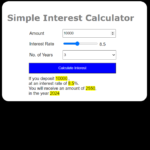## Simple Interest Calculator In JavaScript With Source Code

Simple Interest Calculator is a simple project in HTML5, CSS, and JavaScript. This is an amazing calculator made for the user convenient, to help them calculate out the total amount of interest to the principal sum of a loan or deposit. This project is fo...

Type : Project

File Size : 2.0 KB# Similar Projects and Reports

#### Simple Interest Calculator In JavaScript With Source Code

Simple Interest Calculator is a simple project in HTML5, CSS, and JavaScript. This is an amazing calculator made for the user convenient, to help them calculate out the total amount of interest to the principal sum of a loan or deposit. This project is fo...

#### SIMPLE CALCULATOR IN JAVASCRIPT WITH SOURCE CODE

Simple Calculator is a simple project in HTML5, CSS, and JavaScript. This project is for solving the mathematical calculations of numbers. You can see the basic features of the calculator in this project.

#### Simple JavaScript Calculator With Source Code

This Simple JavaScript calculator project is a simple project in HTML5, CSS, and JavaScript. Here, the calculator functions as that of a real-life simple calculator. This project is for solving the mathematical calculations of numbers. You can see the bas...

#### Simple Tip Calculator In JavaScript With Source Code

The Simple Tip Calculator is a simple project developed using JavaScript, CSS, and HTML. This project is an interesting project. The user can calculate the tip amount that they can get from a single bill pay. Also, the user can divide the tips into number...

#### Simple BMI Calculator In JavaSCript with Source code

Simple BMI Calculator is a simple project developed using HTML5, CSS, and JavaScript. This project is for checking your BMI. With the BMI value, you can check whether you have a healthy weight or not. Also, you can see the basic features of this calculato...

#### Simple Tips Calculator In JavaScript With Source Code

The Simple Tips Calculator is a simple project developed using JavaScript, CSS, and HTML. This project is an interesting project. The user can calculate the tip amount that they can get from a single bill pay. Also, the user can divide the tips into numbe...